Processing ......FreeComputerBooks.com Free Computer, Mathematics, Technical Books and Lecture Notes, etc.

The R Programming LanguageRelated Book Categories:
 Data Analysis and Data Mining, Big Data Neural Networks & Deep LearningData ScienceFinancial Mathematics and Engineering
•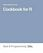Cookbook for R: Best R Programming TIPs (Winston Chang)

The goal of this cookbook is to provide solutions to common tasks and problems in analyzing data. Each recipe tackles a specific problem with a solution you can apply to your own project, and includes a discussion of how and why the recipe works.

•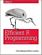Efficient R Programming: Practical Guide to Smarter Programming

This book is about increasing the amount of work you can do with R in a given amount of time. It's about both computational and programmer efficiency. This book is for anyone who wants to make their use of R more reproducible, scalable, and faster.

•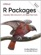R Packages: Organize, Test, Document, and Share Your Code

Turn your R code into packages that others can easily download and use. This practical book shows you how to bundle reusable R functions, sample data, and documentation together by applying author's package development philosophy.

•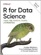R for Data Science: Visualize, Model, Transform, Tidy, & Import

This book teaches you how to do data science with R: You'll learn how to get your data into R, get it into the most useful structure, transform it, visualize it and model it, how data science can help you work with the uncertainty and capture the opportunities.

•Learning Statistics with R (Daniel Navarro)

This book takes you on a guided tour of software development with R, from basic types and data structures to advanced topics like closures, recursion, and anonymous functions. No statistical knowledge is required.

•Text Mining with R: A Tidy Approach (Julia Silge, et al)

You'll explore text-mining techniques with tidytext, a package that authors developed using the tidy principles behind R packages like ggraph and dplyr. You'll learn how tidytext and other tidy tools in R can make text analysis easier and more effective.

•An Introduction to Statistical Learning: with Applications in R

It provides an accessible overview of the field of statistical learning, an essential toolset for making sense of the vast and complex data sets that have emerged in fields ranging from biology to finance to marketing to astrophysics in the past twenty years.

•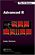Advanced R Programming (Hadley Wickham)

This book presents useful tools and techniques for attacking many types of R programming problems. It not only helps current R users become R programmers but also shows existing programmers what's special about R.

•R for Beginners (Sasha D. Hafner)

The objective of this book is to introduce participants to the use of R for data manipulation and analysis. It is intented for individuals with little or no prior experience in R. The topics that are covered are the most important for getting started with R.

•Just Enough R: Learn Data Analysis with R in a Day (S. Raman)

Learn R programming for data analysis in a single day. The book aims to teach data analysis using R within a single day to anyone who already knows some programming in any other language.

•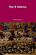The R Inferno (Patrick Burns)

This book is an essential guide to the trouble spots and oddities of R. In spite of the quirks exposed here, R is the best computing environment for most data analysis tasks. If you are using spreadsheets to understand data, switch to R.

•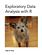Exploratory Data Analysis with R (Roger D. Peng)

This book covers the essential exploratory techniques for summarizing data with R. These techniques are typically applied before formal modeling commences and can help inform the development of more complex statistical models.

•Linear Regression Using R: An Introduction to Data Modeling

This book presents one of the fundamental data modeling techniques in an informal tutorial style. Learn how to predict system outputs from measured data using a detailed step-by-step process to develop, train, and test reliable regression models.

•Analyzing Linguistic Data: Introduction to Statistics using R

A straightforward introduction to the statistical analysis of language, designed for those with a non-mathematical background. Using the leading statistics programme 'R'. Suitable for all those working with quantitative language data.

•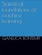Statistical Foundations of Machine Learning using R

This book aims to present the statistical foundations of machine learning intended as the discipline which deals with the automatic design of models from data. All the examples are implemented in the statistical programming language R.

•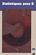Statistics with R (Vincent Zoonekynd)

This book provides an elementary-level introduction to R, targeting both non-statistician scientists in various fields and students of statistics.

•Introduction to Probability and Statistics Using R (G. Jay Kerns)

This is a textbook for an undergraduate course in probability and statistics. Calculus and some linear algebra knowledge is required.

•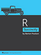R Succinctly (Barton Poulson)

Begin developing your mastery of the powerful R programming language. Become comfortable with the R environment and learn how to find ways for R to fulfill your data needs.

•The Art of R Programming: A Tour of Statistical Software Design

A guided tour of software development with R, from basic types and data structures to advanced topics like closures, recursion, and anonymous functions. No statistical knowledge is required, and your programming skills can range from hobbyist to pro.

•Using R for Data Analysis and Graphics (J H Maindonald)

This book guides users through the practical, powerful tools that the R system provides. The emphasis is on hands-on analysis, graphical display, and interpretation of data.

•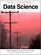Introduction to Data Science with Introduction to R (J. Stanton)

This book provides non-technical readers with a gentle introduction to essential concepts and activities of data science. For more technical readers, the book provides explanations and code for a range of interesting applications using the open source R language for statistical computing and graphics.

•An Introduction to R: A Programming Environment for Data Analysis

This tutorial manual provides a comprehensive introduction to R, an open source software package for statistical computing and graphics

•Introduction to Statistical Thinking, with R, without Calculus

This is an introduction to statistics, with R, without calculus, for students who are required to learn statistics, students with little background in mathematics and often no motivation to learn more.

•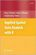Applied Spatial Data Analysis with R (Roger S. Bivand, et al)

This book will be of interest to researchers who intend to use R to handle, visualise, and analyse spatial data.

•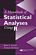A Handbook of Statistical Analyses Using R (Brian S. Everitt, et al)

This book is the perfect guide for newcomers as well as seasoned users of R who want concrete, step-by-step guidance on how to use the software easily and effectively for nearly any statistical analysis.

•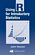Using R for Introductory Statistics (John Verzani)

This book lays the foundation for further study and development in statistics using R - an ideal text for integrating the study of statistics with a powerful computational tool.

•Exploring Data Science (Nina Zumel, et al)

This book introduces readers to various areas in data science and explains which methodologies work best for each, with practical examples in R, Python, and other languages.

•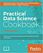Practical Data Science Cookbook using Python and R

Starting with the basics, this book covers how to set up your numerical programming environment, introduces you to the data science pipeline, and guides you through several data projects in a step-by-step format.

•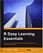R Deep Learning Essentials (Joshua F. Wiley)

This book offers you the essentials every developer needs to get started - from the packages you need to have for your side, building models related to neural networks, prediction, and deep prediction, to fine tuning and optimizing everything you have.

•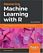Mastering Machine Learning with R (Cory Lesmeister)

This book will teach you advanced techniques in machine learning with the latest code in R. You will delve into statistical learning theory and supervised learning; design efficient algorithms; learn about creating Recommendation Engines; and more.

•The R Programming Language

This is the previous page of The R Programming Language, we are in the processing to convert all the books there to the new page. Please check this page daily!!!

Book CategoriesAll CategoriesRecent BooksIT Research LibraryMiscellaneous Books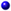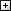Computer LanguagesComputer ScienceData Science/DatabasesElectronic EngineeringJava and Java EE (J2EE)Linux and UnixMathematicsMicrosoft and .NETMobile ComputingNetworking and CommunicationsSoftware EngineeringSpecial TopicsWeb Programming
Other Categories